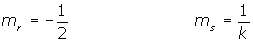Learn from home

The teachersTwo lines are parallel if their slopes are equal.Two lines are parallel if the respective coefficients of x and y are proportional.Two lines are parallel if their directional vectors are equal.Two lines are parallel if the angle between them is 0º.Calculate k so that the lines r ≡ x + 2y − 3 = 0 and s ≡ x − ky + 4 = 0, are parallel.Determine the equation for the line parallel to r ≡ x + 2 y + 3 = 0 that passes through the point A = (3, 5).Determine the equation for the line parallel to r ≡ 3x + 2y − 4 = 0 that passes through the point A = (2, 3).

3 · 2 + 2· 3 + k = 0 k = −12

3x + 2y − 12= 0

The line r ≡ 3x + ny − 7 = 0 passes through the point A = (3, 2) and is parallel to the line s ≡ mx + 2y − 13 = 0. Calculate the values of m and n.Did you like the article?(1 votes, average: 5.00 out of 5)Loading...

Emma

I am passionate about travelling and currently live and work in Paris. I like to spend my time reading, gardening, running, learning languages and exploring new places.

Did you like
this resource?

Bravo!